Home > CC3 > Chapter 9 > Lesson 9.1.3 > Problem9-33

9-33.
1.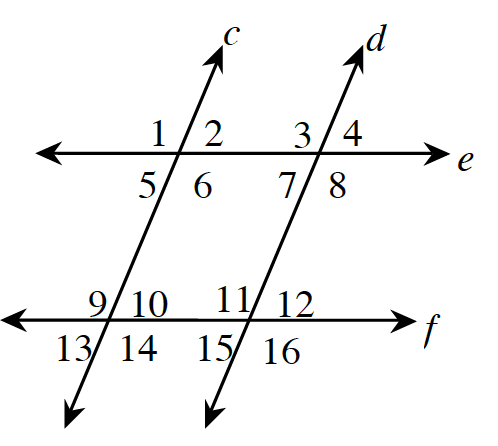Based on the given information, determine which pairs of lines, if any, are parallel. If none are necessarily parallel, write “none.” Homework Help ✎

1. m∠2 = m∠7

2. m∠3 = m∠11

3. m∠1 = m∠12

4. m∠13 = m∠12

5. ∠6 and ∠7 are supplementary.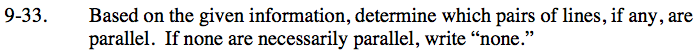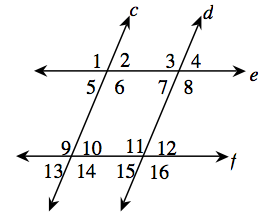Alternate interior angles and corresponding angles are equal only if the lines cut by the transversal are parallel.
Conversely, if the angles are equal the lines must be parallel.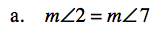These are alternate interior angles.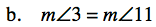These are corresponding angles.

e and f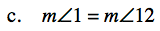In the diagram below, angles 1 and 12 are equal.
Do any two lines look parallel?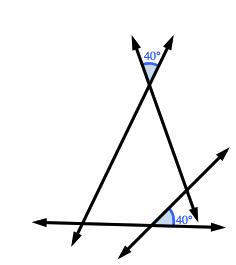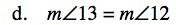Vertical angles are always equal.

c and d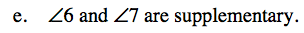Angle 6 would be supplementary to angle 7 if angle 7 was equal to angle 2.